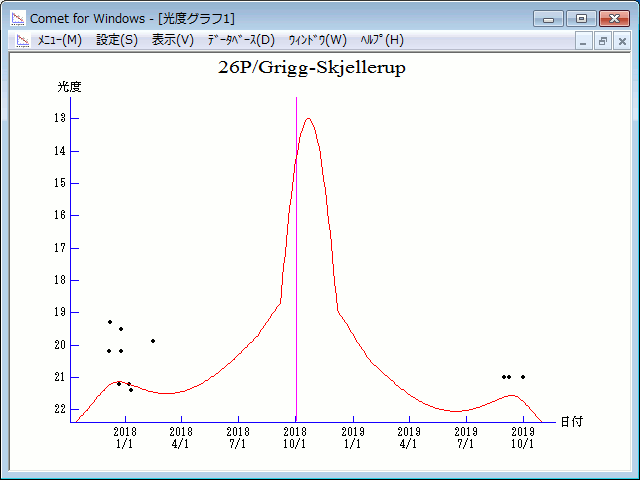# 26P/Grigg-Skjellerup (2018)###Orbital Elements

```Epoch 2018 Oct. 9.0 TT = JDT 2458400.5
T 2018 Oct. 1.82037 TT                                  Kobayashi
q   1.0822949            (2000.0)            P               Q
n   0.18832304     Peri.    2.20048     -0.83314280     +0.51573717
a   3.0143968      Node   211.53392     -0.51307475     -0.85556541
e   0.6409580      Incl.   22.44971     -0.20646399     +0.04497783
P   5.23
From 565 observations 1972 Feb. 12-2014 Sept. 30, mean residual 0".8.
Nongravitational parameters A1 = +0.01, A2 = -0.0010.
```

###Finding Charts###Magnitudes Graph

```        H = 16.5  G = 0.15                         [   ,-60]  (             - 2018 Aug.  2)
m1 = 16.2 + 5 log d +  10.0 log r(t - 20)  [-60,-25]  (2018 Aug.  2 - 2018 Sept. 6)
m1 =  8.0 + 5 log d + 100   log r(t - 20)  [-25, 65]  (2018 Sept. 6 - 2018 Dec.  5)
m1 = 16.2 + 5 log d +  10.0 log r(t - 20)  [ 65,120]  (2018 Dec.  5 - 2019 Jan. 29)
H = 16.5  G = 0.15                         [120,   ]  (2019 Jan. 29 -              )
```##### The orbital elements are published on M.P.E.C. 2015-M77. The charts are made with StellaNavigator Ver.10 (AstroArts). The magnitudes graphs are made with Comet for Windows.